# Project Euler – Problem 75 Solution

Check out my new course Learn you some Lambda best practice for great good! and learn the best practices for performance, cost, security, resilience, observability and scalability.

The problem description is here, and click here to see all my other Euler solutions in F#.

I based my solution on Euclid’s formula for generating Pythagorean triples.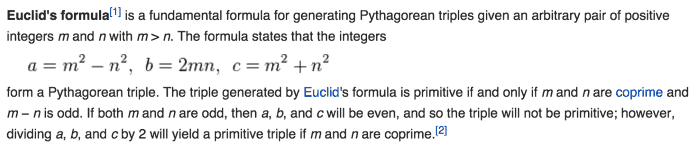And given that max L is 1,500,000, the maximum value for m we need to consider is$\sqrt{\frac{L}{2}}$. Because$L = a + b + c$ and$a^2 + b^2 = c^2$, we can deduce that$c < \frac{L}{2}$; and since$c = m^2 + n^2$ we also have$m < \sqrt{c}$ and therefore$m < \sqrt{\frac{L}{2}}$.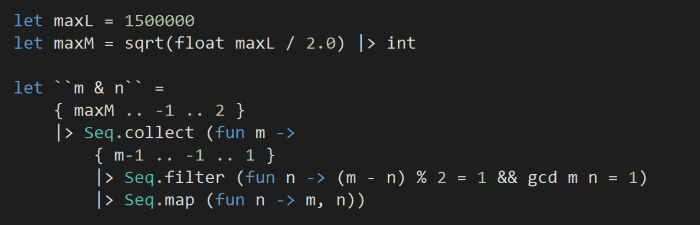The above makes use of a recursive function to calculate the GCD (based on Euclidean Algorithm):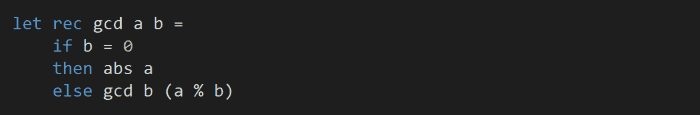For efficiency, we’ll create a cache to store the number of ways L can be used to create integer sided right-angle triangle. As we iterate through the m and n pairs we generated above, we’ll take advantage of the fact that if$a^2 + b^2 = c^2$ then$ka^2 + kb^2 = kc^2$ must also be true and increment multiples of L by one.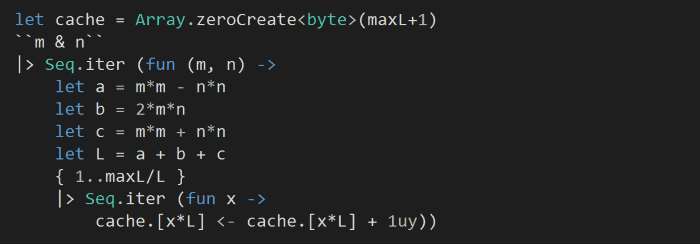Finally, to work out the answer:This solution runs for about 350ms on my machine.

The source code for this solution is here.Hi, I’m Yan. I’m an AWS Serverless Hero and the author of Production-Ready Serverless.

I specialise in rapidly transitioning teams to serverless and building production-ready services on AWS.

Are you struggling with serverless or need guidance on best practices? Do you want someone to review your architecture and help you avoid costly mistakes down the line? Whatever the case, I’m here to help.Check out my new course, Learn you some Lambda best practice for great good! In this course, you will learn best practices for working with AWS Lambda in terms of performance, cost, security, scalability, resilience and observability. Enrol now and enjoy a special preorder price of £9.99 (~\$13).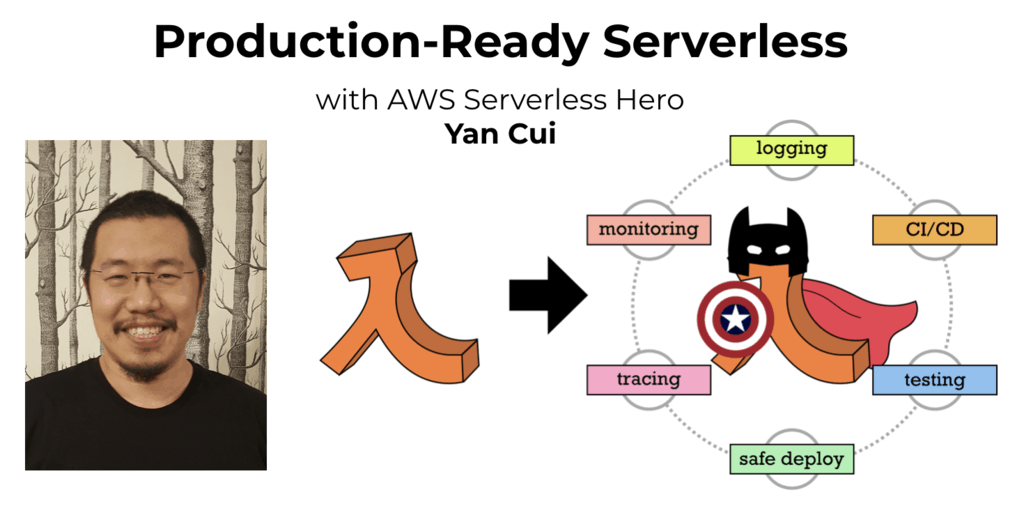Are you working with Serverless and looking for expert training to level-up your skills? Or are you looking for a solid foundation to start from? Look no further, register for my Production-Ready Serverless workshop to learn how to build production-grade Serverless applications!